Chapter 8. Electronic Structure

# Periodic Trends

Learning Objectives

1. Be able to state how certain properties of atoms vary based on their relative position on the periodic table.

One of the reasons the periodic table is so useful is because its structure allows us to qualitatively determine how some properties of the elements vary versus their position on the periodic table. The variation of properties versus position on the periodic table is called . There is no other tool in science that allows us to judge relative properties of a class of objects like this, which makes the periodic table a very useful tool. Many periodic trends are general. There may be a few points where an opposite trend is seen, but there is an overall trend when considered across a whole row or down a whole column of the periodic table.

# Effective Nuclear Charge

Many of the periodic properties of atoms depend on electron configuration; in particular, the valence electrons and their level of attraction to the nucleus.

Valence electrons are simultaneously attracted to the positive charge of the nucleus and screened (repelled) by the negative charges of other electrons. This net nuclear charge felt by valence electrons is known as its , Zeff (pronounced “zed-effective”). The effective nuclear charge is always less than the actual nuclear charge, and can be roughly estimated using the following equation: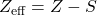Where Z is the nuclear charge (equal to the number of protons), and S is the screening constant which can be approximated to the number of non-valence or “core” electrons.

For example: try to approximate the effective nuclear charge of magnesium.

First, we must determine the electron configuration of magnesium to determine the number of core electrons: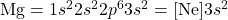Therefore, magnesium has 10 core electrons from its 1s2, 2s2, and 2p6 orbitals.

Magnesium is element 12, so it has 12 protons and a nuclear charge of 12: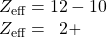Moving left to right across a period on the periodic table, each subsequent element has an additional proton and valence electron, but the core electrons which are responsible for the majority of screening remain the same. This results in a trend that in general the effective nuclear charge increases from left to right across any period of the periodic table.

Moving from top to bottom down a column of the periodic table, we might expect the elements to have a similar effective nuclear charge as they all have the same number of valence electrons. However, we actually see a slight increase in Zeff moving down a column of the periodic table. As the principal quantum number (n) increases, the orbital size increases making the core electron clouds more spread out. These core electron clouds that are more diffuse do not screen as well, giving a slight increase to Zeff (see Figure 8.23 “Increasing Effective Nuclear Charge”).

The  is an indication of the size of an atom. Although the concept of a definite radius of an atom is a bit fuzzy, atoms behave as if they have a certain radius. Such radii can be estimated from various experimental techniques, such as the x-ray crystallography of crystals.

As you go down a column of the periodic table, the atomic radii increase. This is because the valence electron shell is getting a larger and there is a larger principal quantum number, so the valence shell lies physically farther away from the nucleus. This trend can be summarized as follows: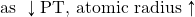Where PT stands for “periodic table.” Going across a row on the periodic table, left to right, the trend is different. This is because although the valence shell maintains the same principal quantum number, the number of protons — and hence the nuclear charge — is increasing as you go across the row. The increasing positive charge leads to a larger effective nuclear charge which casts a tighter grip on the valence electrons, so as you go across the periodic table, the atomic radii decrease. Again, we can summarize this trend as follows: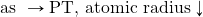Figure 8.24 “Atomic Radii Trends on the Periodic Table” shows spheres representing the atoms of the s and p blocks from the periodic table to scale, showing the two trends for the atomic radius.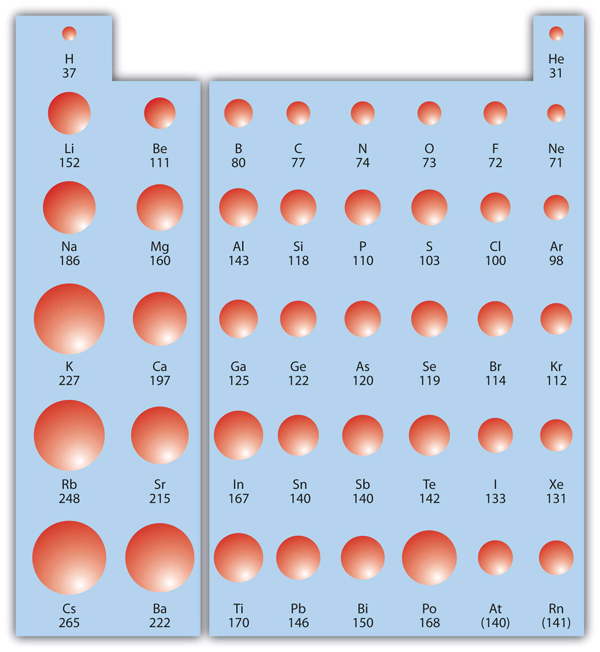Figure 8.24 “Atomic Radii Trends on the Periodic Table.” Although there are some reversals in the trend (e.g., see Po in the bottom row), atoms generally get smaller as you go across the periodic table and larger as you go down any one column. Numbers are the radii in pm. [Long Description]

Example 8.9

# Problems

Referring only to a periodic table and not to Figure 8.24 “Atomic Radii Trends on the Periodic Table,” which atom is larger in each pair?

1. Si or S
2. S or Te

## Solutions

1. Si is to the left of S on the periodic table, so it is larger because as you go across the row, the atoms get smaller.
2. S is above Te on the periodic table, so Te is larger because as you go down the column, the atoms get larger.

# Test Yourself

Referring only to a periodic table and not to Figure 8.24 “Atomic Radii Trends on the Periodic Table,” which atom is smaller, Ca or Br?

Br

# Ionization Energy

is the amount of energy required to remove an electron from an atom in the gas phase: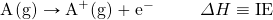IE is usually expressed in kJ/mol of atoms. It is always positive because the removal of an electron always requires that energy be put in (i.e., it is endothermic). IE also shows periodic trends. As you go down the periodic table, it becomes easier to remove an electron from an atom (i.e., IE decreases) because the valence electron is farther away from the nucleus. Thus: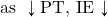However, as you go across the periodic table and the electrons get drawn closer in, it takes more energy to remove an electron; as a result, IE increases: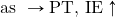Figure 8.25 “Ionization Energy Trends on the Periodic Table” shows values of IE versus position on the periodic table. Again, the trend isn’t absolute, but the general trends going across and down the periodic table should be obvious.

IE also shows an interesting trend within a given atom. This is because more than one IE can be defined by removing successive electrons (if the atom has them to begin with):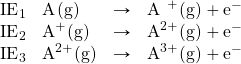And so forth.

Each successive IE is larger than the previous because an electron is being removed from an atom with a progressively larger positive charge. However, IE takes a large jump when a successive ionization goes down into a new shell. For example, the following are the first three IEs for Mg, whose electron configuration is 1s22s22p63s2: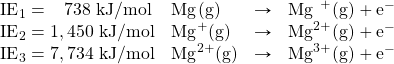The second IE is twice the first, which is not a surprise: the first IE involves removing an electron from a neutral atom, while the second one involves removing an electron from a positive ion. The third IE, however, is over five times the previous one. Why is it so much larger? Because the first two electrons are removed from the 3s subshell, but the third electron has to be removed from the n = 2 shell (specifically, the 2p subshell, which is lower in energy than the n = 3 shell). Thus, it takes much more energy than just overcoming a larger ionic charge would suggest. It is trends like this that demonstrate that electrons are organized in atoms in groups.

Example 8.10

# Problems

Which atom in each pair has the larger IE?

1. Ca or Sr
2. K or K+

## Solutions

1. Because Sr is below Ca on the periodic table, it is easier to remove an electron from it; thus, Ca has the higher IE.
2. Because K+ has a positive charge, it will be harder to remove another electron from it, so its IE is larger than that of K. Indeed, it will be significantly larger because the next electron in K+ to be removed comes from another shell.

# Test Yourself

Which atom has the lower ionization energy, C or F?

C

# Electron Affinity

The opposite of IE is described by , which is the energy change when a gas-phase atom accepts an electron: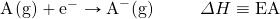EA is also usually expressed in kJ/mol. EA also demonstrates some periodic trends, although they are less obvious than the other periodic trends discussed previously. Generally, as you go across the periodic table, EA increases its magnitude: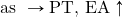There is not a definitive trend as you go down the periodic table; sometimes EA increases, sometimes it decreases. Figure 8.26 “Electron Affinity on the Periodic Table” shows EA values versus position on the periodic table for the s– and p-block elements. The trend isn’t absolute, especially considering the large positive EA values for the second column. However, the general trend going across the periodic table should be obvious.

Example 8.11

# Problems

Predict which atom in each pair will have the highest magnitude of EA.

1. C or F
2. Na or S

## Solutions

1. C and F are in the same row on the periodic table, but F is farther to the right. Therefore, F should have the larger magnitude of EA.
2. Na and S are in the same row on the periodic table, but S is farther to the right. Therefore, S should have the larger magnitude of EA.

# Test Yourself

Predict which atom will have the highest magnitude of EA, As or Br.

Br

Key Takeaways

• Certain properties — notably effective nuclear charge, atomic radius, IE, and EA — can be qualitatively understood by the positions of the elements on the periodic table.

Exercises

# Questions

1. Write a chemical equation with an IE energy change.
2. Write a chemical equation with an EA energy change.
3. State the trends in atomic radii as you go across and down the periodic table.
4. State the trends in IE as you go across and down the periodic table.
5. Which atom of each pair is larger?
1. Na or Cs
2. N or Bi
6. Which atom of each pair is larger?
1. C or Ge
2. Be or Ba
7. Which atom of each pair is larger?
1. K or Cl
2. Ba or Bi
8. Which atom of each pair is larger?
1. Si or S
2. H or He
9. Which atom has the higher IE?
1. Na or S
2. Ge or Br
10. Which atom has the higher IE?
1. C or Ne
2. Rb or I
11. Which atom has the higher IE?
1. Li or Cs
2. Se or O
12. Which atom has the higher IE?
1. Al or Ga
2. F or I
13. A third-row element has the following successive IEs: 738; 1,450; 7,734; and 10,550 kJ/mol. Identify the element.
14. A third-row element has the following successive IEs: 1,012; 1,903; 2,912; 4,940; 6,270; and 21,300 kJ/mol. Identify the element.
15. For which successive IE is there a large jump in IE for Ca?
16. For which successive IE is there a large jump in IE for Al?
17. Which atom has the greater magnitude of EA?
1. C or F
2. Al or Cl
18. Which atom has the greater magnitude of EA?
1. K or Br
2. Mg or S

1.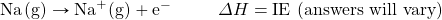1. As you go across, atomic radii decrease; as you go down, atomic radii increase.
1. Cs
2. Bi
1. K
2. Ba
1. S
2. Br
1. Li
2. O
1. Mg
1. The third IE shows a large jump in Ca.
1. F
2. Cl

# Long Descriptions

Figure 8.24 description: Chart of atomic radii trends on the periodic table. The data has been compiled in the following table:

Table 8.5 Atomic Radii Trends on the Periodic Table
First H 37
First He 31
Second Li 152
Second Be 111
Second B 80
Second C 77
Second N 74
Second O 73
Second F 72
Second Ne 71
Third Na 186
Third Mg 160
Third Al 143
Third Si 118
Third P 110
Third S 103
Third Cl 100
Third Ar 98
Fourth K 227
Fourth Ca 197
Fourth Ga 125
Fourth Ge 122
Fourth As 120
Fourth Se 119
Fourth Br 114
Fourth Kr 112
Fifth Rb 248
Fifth Sr 215
Fifth In 167
Fifth Sn 140
Fifth Sb 140
Fifth Te 142
Fifth I 133
Fifth Xe 131
Sixth Cs 265
Sixth Ba 222
Sixth Ti 170
Sixth Pb 146
Sixth Bi 150
Sixth Po 168
Sixth At (140)
Sixth Rn (141)

Figure 8.25 description: Chart of ionization energy on the periodic table. The data has been compiled in the following table:

Table 8.6 Ionization Energy on the Periodic Table
Row Element Ionization Energy (kJ/mol)
First H 1312
First He 2372
Second Li 520
Second Be 111
Second B 800
Second C 1086
Second N 1402
Second O 1214
Second F 1681
Second Ne 2080
Third Na 496
Third Mg 160
Third Al 578
Third Si 786
Third P 1012
Third S 1000
Third Cl 1251
Third Ar 1520
Fourth K 419
Fourth Ca 197
Fourth Ga 579
Fourth Ge 762
Fourth As 946
Fourth Se 940
Fourth Br 1140
Fourth Kr 1350
Fifth Rb 403
Fifth Sr 550
Fifth In 558
Fifth Sn 708
Fifth Sb 833
Fifth Te 870
Fifth I 1008
Fifth Xe 1170
Sixth Cs 376
Sixth Ba 503
Sixth Ti 590
Sixth Pb 715
Sixth Bi 703
Sixth Po 812
Sixth At 800
Sixth Rn 1040

Figure 8.26 description: Chart of electron affinity on the periodic table. The data has been compiled in the following table:

Table 8.7 Electron Affinity on the Periodic Table
Row Element Electron Affinity (kJ/mol)
First H −73
Second Li −60
Second Be 241
Second B −27
Second C −122
Second N ~0
Second O −141
Second F −328
Third Na −53
Third Mg 230
Third Al −43
Third Si −134
Third P −72
Third S −200
Third Cl −349
Fourth K −48
Fourth Ca 156
Fourth Ga −29
Fourth Ge −119
Fourth As −78
Fourth Se −195
Fourth Br −325
Fifth Rb −47
Fifth Sr 167
Fifth In −29
Fifth Sn −107
Fifth Sb −103
Fifth Te −190
Fifth I −295
Sixth Cs −46
Sixth Ba 52
Sixth Ti −19
Sixth Pb −35
Sixth Bi −35